International
Tables for
Crystallography
Volume B
Reciprocal space
Edited by U. Shmueli

International Tables for Crystallography (2006). Vol. B. ch. 2.5, pp. 318-319   | 1 | 2 |

## Section 2.5.6.7. Reconstruction using Fourier transformation

B. K. Vainshteinc

#### 2.5.6.7. Reconstruction using Fourier transformation

| top | pdf |

This method is based on the Fourier projection theorem [(2.5.6.3)–(2.5.6.5)]. The reconstruction is carried out according to scheme (2.5.6.6)(DeRosier & Klug, 1968; Crowther, DeRosier & Klug, 1970; Crowther, Amos et al. 1970; DeRosier & Moore, 1970; Orlov, 1975). The three-dimensional Fourier transform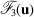is found from a set of two-dimensional cross sections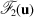on the basis of the Whittaker–Shannon interpolation. If the object has helical symmetry (which often occurs in electron microscopy of biological objects, e.g. on investigating bacteriophage tails, muscle proteins) cylindrical coordinates are used. Diffraction from such structures with c periodicity and scattering density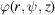is defined by the Fourier–Bessel transform: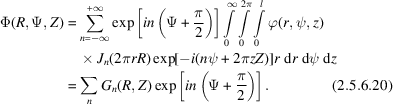The inverse transformation has the form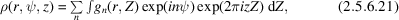so that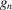and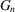are the mutual Bessel transforms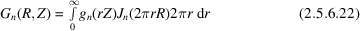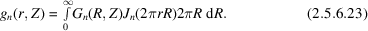Owing to helical symmetry, (2.5.6.22), (2.5.6.23)contain only those of the Bessel functions which satisfy the selection rule (Cochran et al., 1952)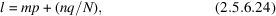where N, q and p are the helix symmetry parameters,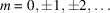. Each layer l is practically determined by the single functionwith the lowest n; the contribution of other functions is neglected. Thus, the Fourier transformation of one projection of a helical structure, with an account of symmetry and phases, gives the three-dimensional transform (2.5.6.23). We can introduce into this transform the function of temperature-factor type filtering the `noise' from large spatial frequencies.

### ReferencesCochran, W., Crick, F. H. C. & Vand, V. (1952). The structure of synthetic polypeptides. 1. The transform of atoms on a helix. Acta Cryst. 5, 581–586.Google ScholarCrowther, R. A., Amos, L. A., Finch, J. T., DeRosier, D. J. & Klug, A. (1970). Three dimensional reconstruction of spherical viruses by Fourier synthesis from electron micrographs. Nature (London), 226, 421–425.Google ScholarCrowther, R. A., DeRosier, D. J. & Klug, A. (1970). The reconstruction of a three-dimensional structure from projections and its application to electron microscopy. Proc. R. Soc. London Ser. A, 317, 319–340.Google ScholarDeRosier, D. J. & Klug, A. (1968). Reconstruction of three dimensional structures from electron micrographs. Nature (London), 217, 130–134.Google ScholarDeRosier, D. J. & Moore, P. B. (1970). Reconstruction of three-dimensional images from electron micrographs of structure with helical symmetry. J. Mol. Biol. 52, 355–369.Google ScholarOrlov, S. S. (1975). Theory of three-dimensional reconstruction. II. The recovery operator. Sov. Phys. Crystallogr. 20, 429–433.Google Scholar